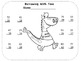# Regrouping Practice Sheet FreebiesSubject
Resource Type
File Type
PDF (9 MB|6 pages)
Standards
FREE
• Product Description
• Standards
Are you tired of the same old regrouping practice sheets? Put a little fun into your students' practice with these three practice sheet freebies. There is one regrouping subtraction sheet each for tens, hundreds and thousands. Each sheet has a grading key.;

Each sheet has 12 problems, which is enough to reinforce the concept, but not enough to overwhelm students. The opportunity to color after the computation is done, helps to ease the stress that often comes with regrouping.

The resource addresses Common Core standards: 2.NBT.B.5, 2.NBT.B.7, 3.NBT.A.2, 4.NBT.B.4

These freebies are taken from a larger resource called "Dragon Eggs: A Regrouping Math Story Unit. To check it out go to:
Dragon Eggs-A Regrouping Math Story Unit

To receive 1/2 off on all future resources during the first 48 hours of launch, be sure and follow me at the green star above.
Fluently add and subtract multi-digit whole numbers using the standard algorithm.
Fluently add and subtract within 1000 using strategies and algorithms based on place value, properties of operations, and/or the relationship between addition and subtraction.
Add and subtract within 1000, using concrete models or drawings and strategies based on place value, properties of operations, and/or the relationship between addition and subtraction; relate the strategy to a written method. Understand that in adding or subtracting three-digit numbers, one adds or subtracts hundreds and hundreds, tens and tens, ones and ones; and sometimes it is necessary to compose or decompose tens or hundreds.
Fluently add and subtract within 100 using strategies based on place value, properties of operations, and/or the relationship between addition and subtraction.
Total Pages
6 pages